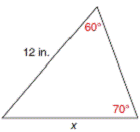Chapter 11.4, Problem 17EElementary Geometry For College St...

7th Edition
Alexander + 2 others
ISBN: 9781337614085

Solutions

Chapter
SectionElementary Geometry For College St...

7th Edition
Alexander + 2 others
ISBN: 9781337614085
Textbook Problem

In Exercises 17 to 22, use a form of the Law of Sines to find the measure of the indicated side or angle. Angle measures should be found to the nearest degree and lengths of sides to the nearest tenth of a unit.To determine

To find:

The measure of the indicates side or angle.

Explanation

Formula:

The Law of Sines,

sinαa=sinβb=sinγc

Where α, β, and γ is the acute angle of the triangle, and a, b, and c is the sides of the triangle.

Calculation:

Given,

Here, take a=12 in.

b=x

β=60°

α=70°

By the Law of Sines,

sinαa=sinβb=sinαc

Take,

sinαa=sinβb

Here,

a=12 in, b=x, β=60°, and α=70°

sin70°12=sin60°x

0

Still sussing out bartleby?

Check out a sample textbook solution.

See a sample solution

The Solution to Your Study Problems

Bartleby provides explanations to thousands of textbook problems written by our experts, many with advanced degrees!

Get Started

In Exercises 11-16, find the slope of the line that passes through each pair of points. 12. (4, 5) and (3, 8)

Applied Calculus for the Managerial, Life, and Social Sciences: A Brief Approach

How are dot products useful?

Calculus: Early Transcendentals

Differentiate. y=x2+1x31

Single Variable Calculus: Early Transcendentals

Add or subtract as indicated. 180(12017)

Trigonometry (MindTap Course List)